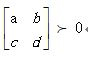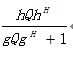# How to express this problem in CVX

Question:
first questiona,b,c,d are four different numbers,I don’t know how to express it in a constraint.

second question:Q is variable,h and g are row vectors,My expression is`h*Q*h/(1+g*Q*g)`,The error shows “Disciplined convex programming error:
Cannot perform the operation: {real affine} ./ {real affine}“

I need your help. If it is convenient for you, please help me. I am looking forward to your reply.

If `b` is not necessarily equal to `c`, this is just an elementwise inequality, which can be entered as [a b;c d] >= 0` or perhaps a small positive number such as 1e-5 instead of zero. CVX treats all inequalities as non-strict.

If this is meant to be symmetric matrix and a semidefinite constraint, follow the guidance at http://cvxr.com/cvx/doc/sdp.html . .

As for the 2nd question, you haven’t shown how the expression will be used. Look up `linear-fractional` at https://web.stanford.edu/~boyd/cvxbook/ .

And the first thing to do is to read the entire CVX User’s Guide, as well as

First of all, thank you for your reply. My second question is that the expression given is the objective function, and I want to get the minimum value of the expression. When I express it as’ H Q H /(1+ G Q G) ',The error shows “Disciplined convex programming error:Cannot perform the operation: {real affine} ./ {real affine}“，Q is semidefinite matrix，I don’t know what this formula is in terms of CVX

Did you read the links?